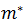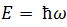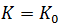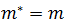# Effective mass - Engineering Physics

Effective mass

An electron in a crystal interacts with the lattice potential, hence its response to external force is different from a free electron. Hence the deviation of electrons behavior is taken into account by considering electrons to have effective mass.

It can be negative and positive or infinite. It is given by

Let us consider an electron in external electric field E. when this field acts on electron for a small time dt, electron gains some energy

According to de-Broglie theory, the velocity of an electron with wave vector K is the same as group velocity of wave representing the electron i.e.

is the angular frequency and is related to the electron energy E by the relation. In term of energy, the velocity is expressed as

Therefore by using eq. (2) in eq. (1), we get

Now

Hence to get acceleration, differentiating this eq. w.r.t

From eq. (3) and (4), we get

Comparing this result with classical result

It follows that an electron behaves as if it has an effective massis given by

In fig.is represented as a function of . An interesting fact we observe here is thatis positive in the lower half and negative in the upper half of the energy band andbecome infinite at the inflection point. This means that in the upper half the electron behaves as a positively charged particle which is usually referred to as a hole. In most conductorsbecause the band is only partially filled. But in semiconductors and insulators having almost filled valence bands, the effective mass plays an important role in the conduction process.

1.